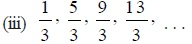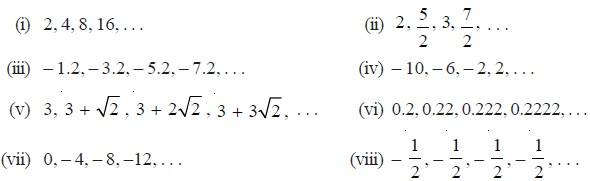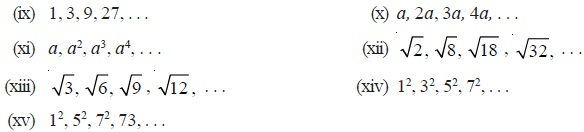Study Materials

NCERT Solutions for Class 10th Mathematics

Page 1 of 4

Chapter 5. Arithmetic Progressions

Exercise 5.1

Exercise 5.1

Q1. In which of the following situations, does the list of numbers involved make an arithmetic progression, and why?
(i) The taxi fare after each km when the fare is Rs 15 for the first km and Rs 8 for each additional km.
(ii) The amount of air present in a cylinder when a vacuum pump removes 1/4 of the air remaining in the cylinder at a time.
(iii) The cost of digging a well after every metre of digging, when it costs Rs 150 for the first metre and rises by Rs 50 for each subsequent metre.
(iv) The amount of money in the account every year, when Rs 10000 is deposited at compound interest at 8 % per annum.

Q2. Write first four terms of the AP, when the first term a and the common difference d are given as follows:

(i) a = 10, d = 10 (ii) a = –2, d = 0
(iii) a = 4, d = – 3 (iv) a = – 1, d = 1/2
(v) a = – 1.25, d = – 0.25

Q3. 3. For the following APs, write the first term and the common difference:
(i) 3, 1, – 1, – 3, . . . (ii) – 5, – 1, 3, 7, . . .(iv) 0.6, 1.7, 2.8, 3.9, . . .

Q4. Which of the following are APs ? If they form an AP, find the common difference d and write three more terms.Page 1 of 4

Chapter Contents: